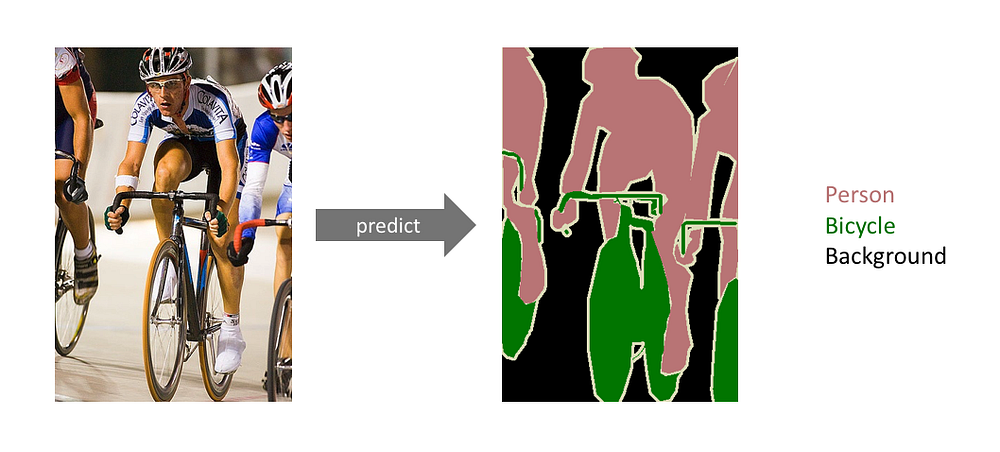# Using Deep Learning Semantic Segmentation method for Ship detection on Satellite Optical Imagery

Source: Deep Learning on Medium# Let’s get down to the Machine Learning script

## First, import all necessary libraries and create the working directories

`import osimport numpy as np # linear algebraimport pandas as pd # data processing, CSV file I/O (e.g. pd.read_csv)from skimage.io import imreadimport matplotlib.pyplot as pltfrom skimage.segmentation import mark_boundariesfrom skimage.util import montageimport gc; gc.enable() # memory is tightfrom skimage.morphology import labelmontage_rgb = lambda x: np.stack([montage(x[:, :, :, i]) for i in range(x.shape)], -1)ship_dir = '../input/airbus-ship-detection'train_image_dir = os.path.join(ship_dir, 'train_v2')test_image_dir = os.path.join(ship_dir, 'test_v2')`

`masks = pd.read_csv(os.path.join('../input/airbus-ship-detection/', 'train_ship_segmentations_v2.csv'))print(masks.shape, 'masks found')#output231723 masks found`

## After that, I can split the data into the training set and validation set.

`from sklearn.model_selection import train_test_splittrain_ids, valid_ids = train_test_split(unique_img_ids,  test_size = 0.3,  stratify = unique_img_ids['ships'])train_df = pd.merge(masks, train_ids)valid_df = pd.merge(masks, valid_ids)print(train_df.shape, 'training masks')print(valid_df.shape, 'validation masks')#output161048 training masks69034 validation maskstrain_df['ships'].hist(bins=np.arange(16)`

Since we grouped our data set by imageId and aggregated their binary value no_ships=0 and ships=1. This histogram depicts on the x-axis the number of aggregated ships per imageId. We can observe a majority of images without ships and a normal decreasing distribution of unique imageId with a least one ship.

Therefore, we need to have a better-balanced training dataset.

Undersample the empty images to get a better-balanced group classes

This code is filtering more the class without ships to create a uniform distribution.

`train_df['grouped_ship_count'] = train_df['ships'].map(lambda x: (x+1)//2).clip(0, 7)#floor div // rounds the result down to the nearest whole number#train_df['grouped_ship_count'].hist() #clip() sets min and max intervals.def sample_ships(in_df, base_rep_val=1500): if in_df['ships'].values==0: return in_df.sample(base_rep_val//3) # even more strongly undersample no ships else: return in_df.sample(base_rep_val, replace=(in_df.shape<base_rep_val))balanced_train_df = train_df.groupby('grouped_ship_count').apply(sample_ships)balanced_train_df['ships'].hist(bins=np.arange(16)) #with 10 bins`

## U-Net Model Design

The architecture looks like a ‘U’ which justifies its name. This architecture consists of three sections: The contraction, The bottleneck, and the expansion section. But the heart of this architecture lies in the expansion section. This action would ensure that the features that are learned while contracting the image will be used to reconstruct it.

`from keras import models, layers# Build U-Net modeldef upsample_conv(filters, kernel_size, strides, padding): return layers.Conv2DTranspose(filters, kernel_size, strides=strides, padding=padding)def upsample_simple(filters, kernel_size, strides, padding): return layers.UpSampling2D(strides)if UPSAMPLE_MODE=='DECONV': upsample=upsample_convelse: upsample=upsample_simpleinput_img = layers.Input(t_x.shape[1:], name = 'RGB_Input')pp_in_layer = input_imgif NET_SCALING is not None: pp_in_layer = layers.AvgPool2D(NET_SCALING)(pp_in_layer)pp_in_layer = layers.GaussianNoise(GAUSSIAN_NOISE)(pp_in_layer)pp_in_layer = layers.BatchNormalization()(pp_in_layer)c1 = layers.Conv2D(8, (3, 3), activation='relu', padding='same') (pp_in_layer)c1 = layers.Conv2D(8, (3, 3), activation='relu', padding='same') (c1) #Going Downp1 = layers.MaxPooling2D((2, 2)) (c1) #2x2 kernelc2 = layers.Conv2D(16, (3, 3), activation='relu', padding='same') (p1)c2 = layers.Conv2D(16, (3, 3), activation='relu', padding='same') (c2)p2 = layers.MaxPooling2D((2, 2)) (c2)c3 = layers.Conv2D(32, (3, 3), activation='relu', padding='same') (p2)c3 = layers.Conv2D(32, (3, 3), activation='relu', padding='same') (c3)p3 = layers.MaxPooling2D((2, 2)) (c3)c4 = layers.Conv2D(64, (3, 3), activation='relu', padding='same') (p3)c4 = layers.Conv2D(64, (3, 3), activation='relu', padding='same') (c4)p4 = layers.MaxPooling2D(pool_size=(2, 2)) (c4)c5 = layers.Conv2D(128, (3, 3), activation='relu', padding='same') (p4) #Bottle Neckc5 = layers.Conv2D(128, (3, 3), activation='relu', padding='same') (c5)u6 = upsample(64, (2, 2), strides=(2, 2), padding='same') (c5) #Going Upu6 = layers.concatenate([u6, c4])c6 = layers.Conv2D(64, (3, 3), activation='relu', padding='same') (u6)c6 = layers.Conv2D(64, (3, 3), activation='relu', padding='same') (c6)u7 = upsample(32, (2, 2), strides=(2, 2), padding='same') (c6)u7 = layers.concatenate([u7, c3])c7 = layers.Conv2D(32, (3, 3), activation='relu', padding='same') (u7)c7 = layers.Conv2D(32, (3, 3), activation='relu', padding='same') (c7)u8 = upsample(16, (2, 2), strides=(2, 2), padding='same') (c7)u8 = layers.concatenate([u8, c2])c8 = layers.Conv2D(16, (3, 3), activation='relu', padding='same') (u8)c8 = layers.Conv2D(16, (3, 3), activation='relu', padding='same') (c8)u9 = upsample(8, (2, 2), strides=(2, 2), padding='same') (c8)u9 = layers.concatenate([u9, c1], axis=3)c9 = layers.Conv2D(8, (3, 3), activation='relu', padding='same') (u9)c9 = layers.Conv2D(8, (3, 3), activation='relu', padding='same') (c9)d = layers.Conv2D(1, (1, 1), activation='sigmoid') (c9)d = layers.Cropping2D((EDGE_CROP, EDGE_CROP))(d)d = layers.ZeroPadding2D((EDGE_CROP, EDGE_CROP))(d)if NET_SCALING is not None: d = layers.UpSampling2D(NET_SCALING)(d)seg_model = models.Model(inputs=[input_img], outputs=[d])seg_model.summary()`